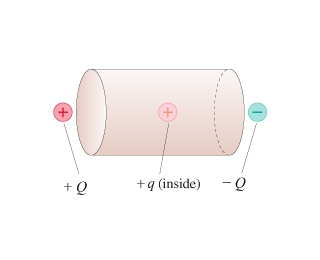# Problem: What is the net electric flux through the cylinder of the figure(Figure 1) ? Assume that Q = 200 nC and q = 4 nC.

###### FREE Expert Solution

We are going to determine the electric flux through the cylinder. We'll use Gauss law.

Gauss law:

$\overline{){{\mathbf{\varphi }}}_{{\mathbf{E}}}{\mathbf{=}}{\mathbf{E}}{\mathbf{A}}{\mathbf{=}}\frac{{\mathbf{Q}}_{\mathbf{e}\mathbf{n}}}{{\mathbf{\epsilon }}_{\mathbf{0}}}}$

We can either use EA or Qen0.

87% (337 ratings)###### Problem Details

What is the net electric flux through the cylinder of the figure(Figure 1) ? Assume that Q = 200 nC and q = 4 nC.Frequently Asked Questions

What scientific concept do you need to know in order to solve this problem?

Our tutors have indicated that to solve this problem you will need to apply the Electric Flux concept. You can view video lessons to learn Electric Flux. Or if you need more Electric Flux practice, you can also practice Electric Flux practice problems.